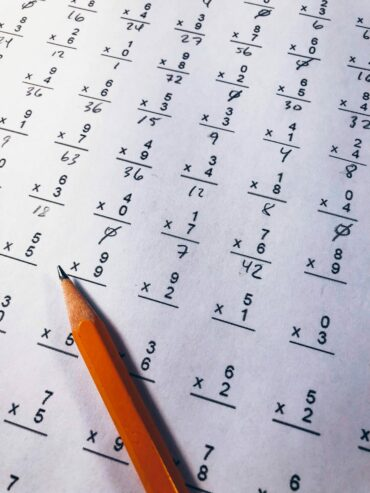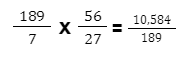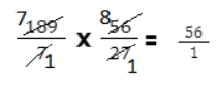# Tips For The Selective School Test Mathematical Reasoning Section

Numerical Reasoning falls under Ability Tests, which test a child’s ability to answer problems without prior information. Ability typically indicates a child’s rate of learning and their capacity to deal with complexity. This article will tackle all about the tips for the selective school test mathematical reasoning section.

Numerical Reasoning is a vital part of other competitive examinations as well. Numerical Reasoning is more difficult than other subjects with higher scores. This is a multiple-choice exam that assesses numerical reasoning and critical thinking. The test questions incorporate series, matrices, mathematical reasoning, and deduction. As such, it extends Mathematics.

Make careful to write down all your calculations instead of calculating them in your mind; without writing them down, pupils are prone to make foolish errors. This also makes it easy for pupils to spot errors and retrace their steps while examining their work. By writing it down, you will be able to reread your train of thought rather of having to start from scratch.

These are the tips and strategies we have for you to improve your score in Mathematical Reasoning Skills:

##### Find the optimal strategy
• Ensure that you are utilizing the most effective approach for each question; otherwise, you will waste time and risk making thoughtless errors!
• Mathematical Reasoning is a multiple-choice part; for more time-consuming and challenging problems, it is sometimes faster to work backwards from the solution via trial and error than to go directly into the question.
##### Remain Calm During the Exam:When taking the Numerical Reasoning test, pupils are overwhelmed with visual, numerical, and written information. The normal response is worry and panic, and they perceive the questions to be absurdly “tough” or “complex.” However, in the vast majority of instances, this is not the case. So, remember to remain composed.

##### There is no "first come, first served" policy:

The majority of students believe that the exam questions are arranged in a descending order of difficulty, with the easiest questions appearing first and the most difficult appearing last.

This is not the situation. Complex questions (category C) may be alternated throughout the exam, i.e. between questions that are relatively simpler (categories A & B).

Therefore, as the exam begins, resist the temptation to immediately answer the first question. Determine which questions fall under category A and answer them first by scanning the full exam. Then, go on to questions from the B category, and if you still have time, the C category.

In countless occasions, a difficult question was placed at the beginning of an exam, causing students to squander valuable time attempting to answer it.

Imagine if a student wasted 10 minutes on one of these difficult questions, leaving him or her with only 20 minutes to complete the other 49 questions. Additionally, attempting easy questions first is a huge morale booster. Students’ confidence grows incrementally when they correctly complete problems in category A.

Confidence is at an all-time low if you have squandered valuable time completing a few problems with dubious results and time appears to be running short.

##### Exclusive Exclusion:

Selective Omission is a strategy that is complementary to the above strategy. The majority of the time should be utilised to identify and solve A & B category of questions in that order.

Omit the questions which are complex, long-winding and heavily worded, at least in the first instance. You may come back to these questions later if time permits.

##### Utilizing Abilities and Problem-Solving Methods:
• Carefully read the question below. Determine what is provided and what is hidden.
• Consider the information: Divide the information into smaller pieces. Separate pertinent information from extraneous information.
• To synthesize pertinent knowledge is to blend distinct pieces to make a unified whole.
• Effective tactics for analysis and synthesis include the use of diagrams, flowcharts, tree diagrams, tables, and the writing of the information.
• Mentally compute as much as possible. Use quick computation techniques.
• Remove the superfluous responses from the supplied options.
• Select the response that corresponds to the given criteria. Oftentimes, in a Quadratic equation situation, it is not necessary to formulate and solve an equation. This will take too much time! Simply check the options that satisfy the stated requirements and pick the correct response.
• Perform exercise alone to perform the required computations.
• Working backwards, guessing and checking, and solving a simplified version of the issue are additional successful methods.
##### Intelligent Estimation:

This is a crucial problem-solving strategy where you may narrow down the answer by eliminating alternatives. This is especially true for category C problems, for which the right response may be determined without actually solving the issue.

##### Attempt All Questions:

Since there is no negative marking on the exam, you should answer every question. You may guess some last answers if necessary. You may get some of these answers right.

##### Time Management:

As previously indicated, the total time allotted for the 50 questions is 30 minutes, or an average of 36 seconds each question. Time management efficiency Focus on your own tasks and avoid becoming sidetracked by those of others.

Lorem ipsum dolor sit amet, consectetur adipiscing elit. Ut elit tellus, luctus nec ullamcorper mattis, pulvinar dapibus leo.

Remember that this is a competitive environment, and you must remain ahead of the pack by adhering to best practices. What matters is how your score compares to those of other students, not your individual score.##### REAL LIFE IS REAL LIFE

Develop a sixth sense for the surrounding actual environment. Unless the car is going downhill, cars do not get 160 miles per gallon, thus if your answer is inconsistent with reality, it is likely incorrect. Unless the test was prepared by a nefarious genius, the real-life questions should be relevant to the world you know. If you arrive at an unusual answer, verify that you have used the right conversion. If a 10-by-15-foot space has a 120-foot ceiling, you may have converted feet to inches incorrectly.

##### CHOOSE THE SIMPLEST CONVERSION

As you read the question, be mindful of the units of measurement. The units in the question should be consistent with the units required in the response. If the question asks for an answer in inches but all the units are in feet, you must convert feet to inches. However, what do you do if some response selections are in feet and others are in inches.

If you must convert units, select the unit with the lowest probability of creating a mistake and the highest probability of predicting the correct result. If a room is 13 by 7 yards and the answer is in feet, it is simple to multiply these figures by 3 in your brain. If the length of the room is 12,48 yards, you would be better off using scratch paper and converting at the end. Whole numbers are more manageable than fractions and decimals.

##### ROUND AND ESTIMATE:

You might be able to choose the right response without having to compute the precise result if the answer options you must choose from differ significantly in magnitude. When a question requiring addition or subtraction is presented, round the numbers to the closest 5 or 10 and perform the calculations mentally. It is simpler to add numbers that finish in 5 or 0 than it is, for instance, to add numbers that end in 3 or 7.

To rapidly establish if the sum is less than the maximum amount if the question includes one, round all values up, as in the following example:

Mary wants to spend no more than $20 while doing some short shopping. She spends$6.73, plus an additional 8% tax, to purchase lipstick, some apples, and grapes. Did she stick to her spending plan?

You can see that $6 +$5 + $7 Equals$18 when all values are rounded up to the next dollar. If the tax rate is 8%, round it up to 10%, then it becomes clear that the tax on $18 would be$1.80. You can see that Mary kept within her means since $18 plus$1.80 is $19.80, which is still less than$20.

Perform your computations after rounding down all other numbers if the question calls for a minimum value.

##### MULTIPLY BY HUNDREDS, TENS AND ONES:

When multiplying a large number by a small one (single- or double-digit number), divide the huge number into its component parts, multiply the parts by the smaller number, then sum the results, as shown below.

76 x 7 = (70 x 7) + (6 x 7) = 490 + 42 = 532

ALWAYS SIMPLIFY:

Before considering multiplying or dividing fractions or conducting any other sequence of mathematical operations, investigate whether the equation may be simplified. By simplifying, you wind up with fewer and smaller numbers and may even be able to solve the arithmetic in your mind, therefore saving valuable exam time. For instance, without simplifyingWhen you simplify:##### DISCOUNTED PRICE COMPUTATION:

If the problem offers a 5% discount from the regular price, you can either multiply the regular price by 0.05 to calculate the discount and subtract that amount from the regular price, or you can calculate that if you’re receiving a 5% discount, you’re paying 95% of the regular price, as 5% of the regular price is 95% as,

100% –  5% = 95%

In place of performing the solution in two stages, you perform it in one step and save a few seconds by multiplying the amount by 0.95 to obtain the same results. You also lessen the likelihood of making a mistake; the more steps you take, the more likely you are to make an error, particularly when you’re in a rush.

I.e:

You are given a 5% discount with the goods you’ve bought, and the total is $100. How much will you pay with a 5% discount?$100 x 0.95 = $95 You will pay$95.

##### ADD THE PERCENTAGE OF TAX OR TIP TO ONE

When calculating tax or tip on a total, you may either compute the tax or tip and add it to the total (two stages) or multiply the tax or tip percentage by the total (one step). In order to give your waiter a 15 percent tip in a restaurant, for instance, you may multiply the amount on the check by 0.15 and add this number to the bill to determine the total tip. Alternatively, you may multiply the check by 1.15 to determine the total including tip (100 percent total plus 15 percent tip is 115 percent , or 1.15). Again, fewer steps and less opportunities for error, particularly if mental calculation is used.

## tutoring services

Click the button below to see all available tutoring programs

## Read Our Latest Blog Posts

### Free Word Wizardry Vocabulary Book

Get the book sent straight to your inbox. Just enter your email below.

### Free Persuasive Writing Book

Get the book sent straight to your inbox. Just enter your email below.

### Free Victorian Selective – Reading Comprehension Book

Get the book sent straight to your inbox. Just enter your email below.

### Free Narrative Writing Book

Get the book sent straight to your inbox. Just enter your email below.